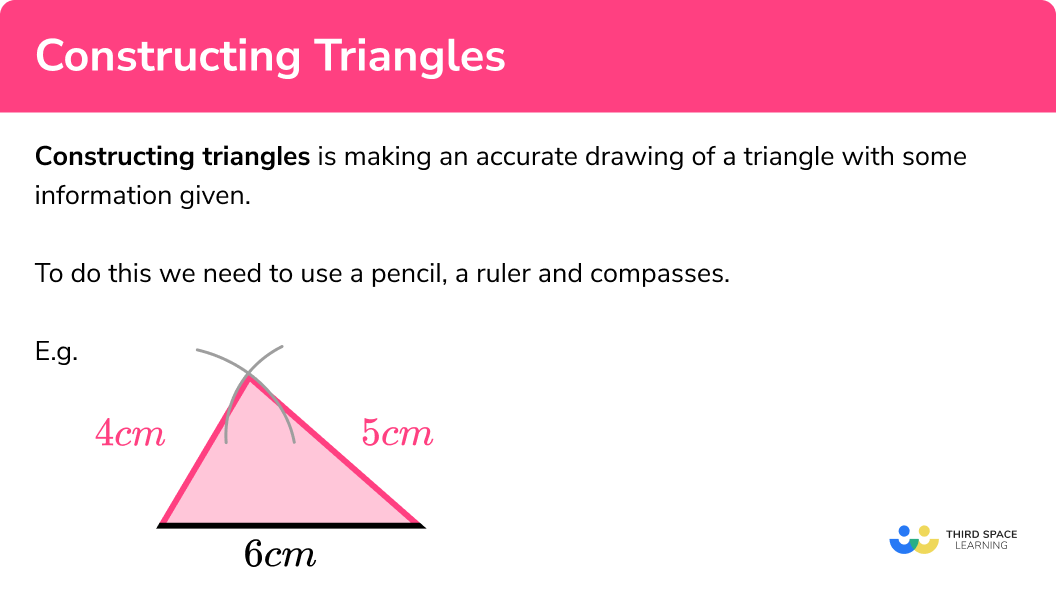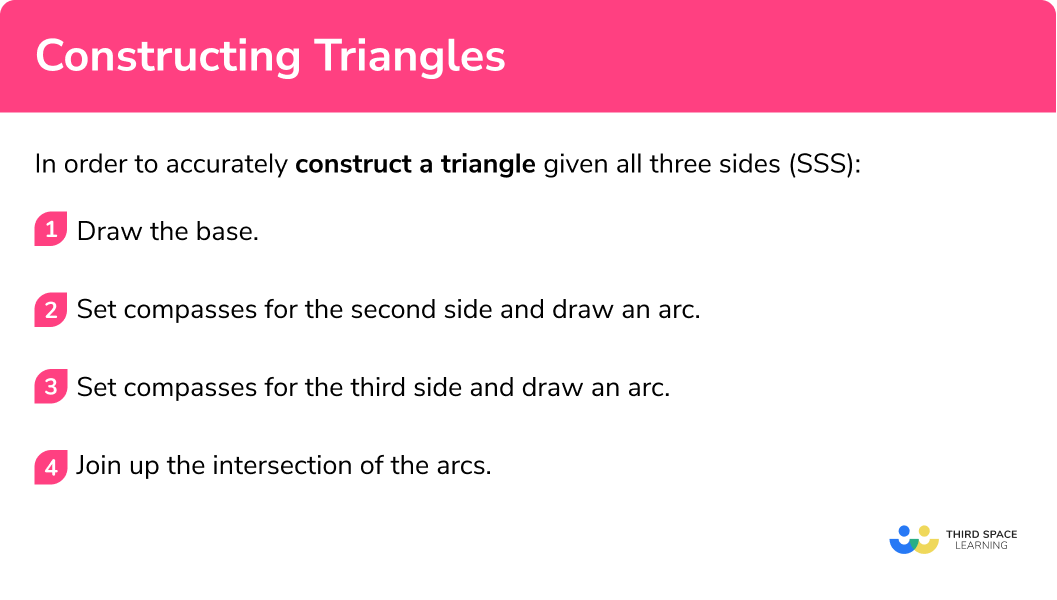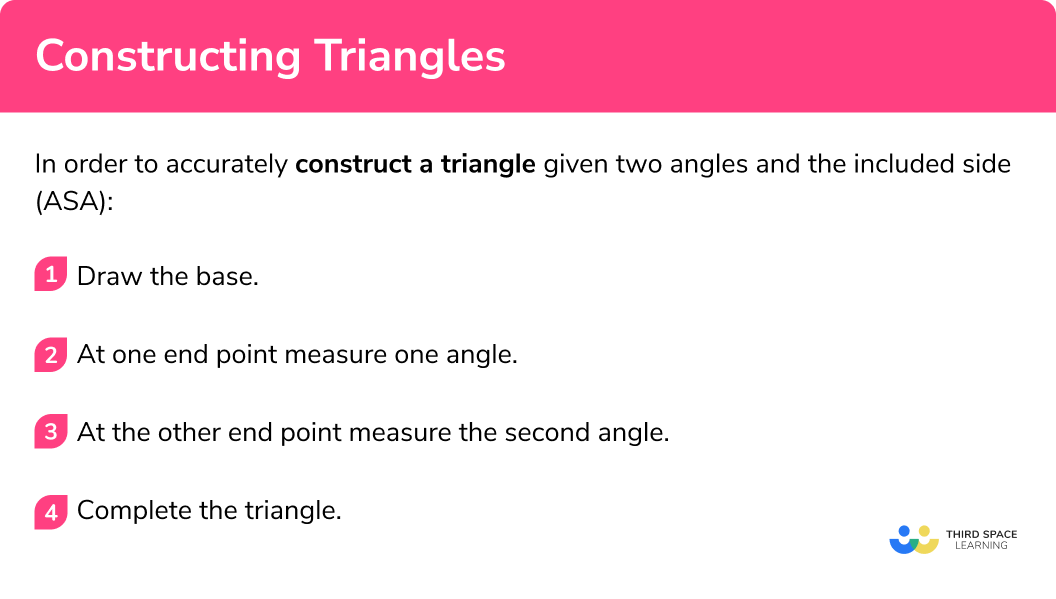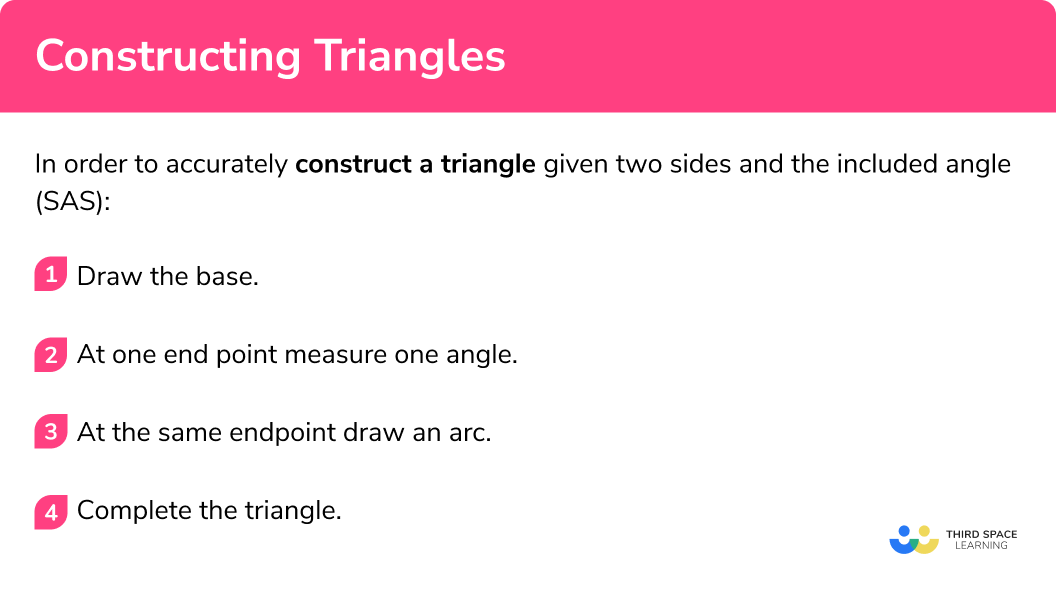# Constructing Triangles

Here we will learn about constructing triangles, including how to accurately draw different triangles using a pencil, a ruler, a protractor and a pair of compasses.

There are also constructions worksheets, including constructing triangles, based on Edexcel, AQA and OCR exam questions, along with further guidance on where to go next if you’re still stuck.

## What is constructing triangles?

Constructing triangles is making an accurate drawing of a triangle with some information given.

To do this we need to use a pencil, a ruler and compasses.

E.g.

Here is a triangle with sides 4cm , 5cm and 6cm constructed using a pencil, a ruler and compasses.

### What is constructing triangles?## How to construct triangles given all three sides

In order to accurately construct a triangle given all three sides (SSS):

1. Draw the base.
2. Set compasses for the second side and draw an arc.
3. Set compasses for the third side and draw an arc.
4. Join up the intersection of the arcs.

### How to construct triangles given all three sides### Related lessons on constructions

Constructing triangles is part of our series of lessons to support revision on construction and loci and construction. You may find it helpful to start with the main loci and construction lesson for a summary of what to expect, or use the step by step guides below for further detail on individual topics. Other lessons in this series include:

## Constructing triangles examples – SSS

### Example 1: All three sides

Draw the triangle ABC accurately

1. Draw the base.

Use a pencil and a ruler to draw the base. It is usually easiest to use the longest side.

2Set compasses for the second side and draw an arc.

Open the compasses to 4cm . Place the point on point A and draw an arc.

3Set compasses for the third side and draw an arc.

Open the compasses to 6cm . Place the point on point C and draw an arc. This second arc should cross the first arc.

4Join up the intersection of the arcs.

Complete the triangle by joining the point where the arcs intersect to point A and point C .

### Example 2: All three sides

Draw the triangle RST accurately

Use a pencil and a ruler to draw the base. It is usually easiest to use the longest side.

Open the compasses to 4.6cm . Place the point on point R and draw an arc.

Open the compasses to 6.2cm . Place the point on point T and draw an arc. This second arc should cross the first arc.

Complete the triangle by joining the point where the arcs intersect to point R and point T .

## How to construct triangles given two angles and the included side

In order to accurately construct a triangle given two angles and the included side (ASA):

1. Draw the base.
2. At one end point measure one angle.
3. At the other end point measure the second angle.
4. Complete the triangle.

### How to construct triangles given two angles and the included side## Constructing triangles examples – ASA

### Example 3: Two angles and the included side

Draw the triangle ABC accurately

Use a pencil and a ruler to draw the base.

At point A use a protractor to measure the angle 50° , make a mark and then draw a straight line from point A through the mark. Make this line long.

At point B use a protractor to measure the angle 30° , make a mark and then draw a straight line from point B though the mark.

Make sure that the two lines interact to form the triangle.

### Example 4: Two angles and the included side

Draw the triangle ABC accurately

Use a pencil and a ruler to draw the base.

At point A use a protractor to measure the angle 25° , make a mark and then draw a straight line from point A through the mark. Make this line long.

At point B use a protractor to measure the angle 63° , make a mark and then draw a straight line from point B though the mark.

Make sure that the two lines interact to form the triangle.

## How to construct triangles given two sides and the included angle

In order to accurately construct a triangle given two sides and the included angle (SAS):

1. Draw the base.
2. At one end point measure one angle.
3. At the same endpoint draw an arc.
4. Complete the triangle.

### How to construct triangles given two sides and the included angle## Constructing triangles examples – SAS

### Example 5: Two sides and the included angle

Draw the triangle ABC accurately

Use a pencil and a ruler to draw the base.

At point B use a protractor to measure the angle 40° , make a mark and then draw a straight line from point B through the mark. Make this line long.

Open the compasses to 5cm . Place the point on point B and draw an arc. This second arc should cross the line drawn in step 2.

Join the intersection of the arc and the line with point A .

### Example 6: Two sides and the included angle

Draw the triangle DEF accurately:

Use a pencil and a ruler to draw the base.

At point E use a protractor to measure the angle 73° , make a mark and then draw a straight line from point E through the mark. Make this line long.

Open the compasses to 5.2cm . Place the point on point B and draw an arc. This second arc should cross the line drawn in step 2.

Join the intersection of the arc and the line with point A .

### Common misconceptions

• The pencil should be sharp

A sharp pencil helps your diagram to be accurate. Using a small pencil in compasses can also be helpful.

• The construction arcs must not be removed

The arcs drawn should be drawn lightly so can be adjusted if needed but they must be visible in your final answer. This is to show that you have used the correct method to draw the triangle accurately.

• Take care when using the protractor to measure angles

Remember to measure angles from 0 .

• If you are given two angles, you can work out the third

If you are given two angles and the side is not included you can work out the third angle by using the fact that the sum of interior angles in a triangle is 180° . Then you can use the two angles and included side detailed above.

### Practice constructing triangles questions

1. Construct a triangle with sides 4cm , 5cm and 6cm .The longest side should be 6cm . Compasses should be used to make the arcs, and they should be clearly seen. The other two sides are of different lengths; 4cm and 5cm .

2. Construct a triangle with sides 7cm , 6cm and 6cm .The longest side should be 7cm . Compasses should be used to make the arcs, and they should be clearly seen. The other two sides are the same length; 6cm . The triangle is an isosceles triangle.

3. Construct a triangle ABC , with AB is 6cm , angle BAC is 45^{\circ} and angle ABC is 60^{\circ} .Check the labelling of the triangle. The side AB should be 6cm . The angle at A should be 45^{\circ} and angle at B should be 60^{\circ} .

4. Construct a triangle PQR , with PQ is 7cm , angle QPR is 40^{\circ} and angle PQR is 45^{\circ} .Check the labelling of the triangle. The side PQ should be 7cm . The angle at P should be 40^{\circ} and angle at Q should be 45^{\circ} .

5. Construct a triangle ABC , with AB is 6cm , BC is 5cm and angle ABC is 60^{\circ} .Check the labelling of the triangle. The side AB should be 6cm and the side BC should be 7cm . The angle at B should be 60^{\circ} .

6. Construct a triangle ABC , with AB is 6cm , BC is 5cm and angle ABC is 60^{\circ} .Check the labelling of the triangle. The side RS should be 7cm and the side ST should be 6cm . The angle at S should be 50^{\circ} .

### Constructing triangles GCSE questions

1. In the space below, use a ruler and compasses to construct an equilateral triangle with sides 7cm .

You must show all your construction lines.

One side of the triangle has already been drawn for you.(2 marks)for the construction arcs

(1)

for the completed equilateral triangle

(1)

2. Here is a triangleMake an accurate drawing of the triangle ABC .

The line AB has already been drawn for you.(2 marks)for the angle B  38-42 degrees OR for the side BC  6.3-6.7cm

(1)

for the completed triangle

(1)

3. Here is a triangleMake an accurate drawing of the triangle ABC .

The line AB has already been drawn for you.(2 marks)for the angle 68-72 degrees OR for the angle 48-52 degrees

(1)

for the completed triangle

(1)

## Learning checklist

You have now learned how to:

• Construct a triangle given all three sides
• Construct a triangle given two angles and the included side
• Construct a triangle given two sides and the included angle

## Still stuck?

Prepare your KS4 students for maths GCSEs success with Third Space Learning. Weekly online one to one GCSE maths revision lessons delivered by expert maths tutors.

Find out more about our GCSE maths tuition programme.